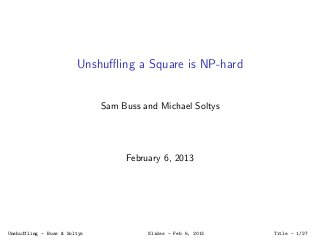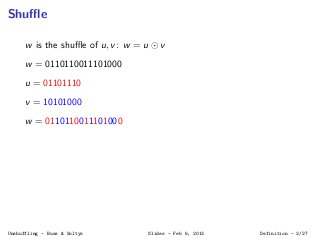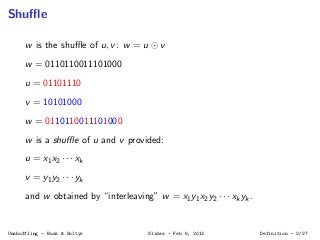Successfully reported this slideshow.

# Unshuffling a square is NP-hard×

1 of 46
1 of 46

# Unshuffling a square is NP-hard

This is a presentation given at the University of California at San Diego on December 3, 2013 (and at McMaster on February 6, 2013) based on the eponymous paper by Sam Buss and Michael Soltys: http://arxiv.org/abs/1211.7161

This is a presentation given at the University of California at San Diego on December 3, 2013 (and at McMaster on February 6, 2013) based on the eponymous paper by Sam Buss and Michael Soltys: http://arxiv.org/abs/1211.7161

### Unshuffling a square is NP-hard

1. 1. Unshuﬄing a Square is NP-hard Sam Buss and Michael Soltys February 6, 2013 Unshuffling - Buss & Soltys Slides - Feb 6, 2013 Title - 1/27
2. 2. Shuﬄe w is the shuﬄe of u, v: w = u v w = 0110110011101000 u = 01101110 v = 10101000 w = 0110110011101000 Unshuffling - Buss & Soltys Slides - Feb 6, 2013 Definition - 2/27
3. 3. Shuﬄe w is the shuﬄe of u, v: w = u v w = 0110110011101000 u = 01101110 v = 10101000 w = 0110110011101000 w is a shuﬄe of u and v provided: u = x1x2 · · · xk v = y1y2 · · · yk and w obtained by “interleaving” w = x1y1x2y2 · · · xkyk . Unshuffling - Buss & Soltys Slides - Feb 6, 2013 Definition - 2/27
4. 4. Square Shuﬄe w is a square provided it is equal to a shuﬄe of a u with itself, i.e., ∃u s.t. w = u u The string w = 0110110011101000 is a square: w = 0110110011101000 and u = 01101100 = 01101100 Unshuffling - Buss & Soltys Slides - Feb 6, 2013 Definition - 3/27
5. 5. Result given an alphabet Σ, |Σ| ≥ 7, Square = {w : ∃u(w = u u)} is NP-complete. What we leave open: What about |Σ| = 2 (for |Σ| = 1, Square is just the set of even length strings) What about if |Σ| = ∞ but each symbol cannot occur more often than, say, 6 times (if each symbol occurs at most 4 times, Square can be reduced to 2-Sat – see P. Austrin Stack Exchange post bit.ly/WATco3) Unshuffling - Buss & Soltys Slides - Feb 6, 2013 Definition - 4/27
6. 6. Bipartite graph w = (c1x3c2)2(c1x2c2)2(c1xc2)2 is a square: w = u u where u = c1xxxc2xc2c1xc1xc2. Unshuffling - Buss & Soltys Slides - Feb 6, 2013 Definition - 5/27
7. 7. Bipartite graph w = (c1x3c2)2(c1x2c2)2(c1xc2)2 is a square: w = u u where u = c1xxxc2xc2c1xc1xc2. Bipartite graph G associated with particular solution w = u u: c1 x x x c2 c1 x x x c2 c1 x x c2 c1 x x c2 c1 x c2 c1 x c2 Unshuffling - Buss & Soltys Slides - Feb 6, 2013 Definition - 5/27
8. 8. Bipartite graph w = (c1x3c2)2(c1x2c2)2(c1xc2)2 is a square: w = u u where u = c1xxxc2xc2c1xc1xc2. Bipartite graph G associated with particular solution w = u u: c1 x x x c2 c1 x x x c2 c1 x x c2 c1 x x c2 c1 x c2 c1 x c2 We also have w = v v with v = c1x3c2c1x2c2c1xc2 which would have its associated bipartite graph Unshuffling - Buss & Soltys Slides - Feb 6, 2013 Definition - 5/27
9. 9. Nesting a b a b a b b a G has the “non-nesting” property: if G contains an edge from wk to w and an edge from wp to wq, then it is not the case that k < p < q < Claim: There is a complete bipartite graph G of degree one (i.e., a perfect matching) on the symbols of w which is non-nesting, iﬀ w can be expressed as a square shuﬄe. Unshuffling - Buss & Soltys Slides - Feb 6, 2013 Definition - 6/27
10. 10. Formal languages Ginsburg & Spanier in ’65 Modelling sequential execution of concurrent processes Riddle (’73 & ’79), Shaw (’78), Hoare Shuﬄe on its own Mansﬁeld (’82 & ’83; polytime dynamic prog. alg. for w = u1 u2 · · · uk for constant k; when k varies, NP-complete) Warmuth & Haussler (’84; show w = u u · · · u NP-complete by reduction from 3-Partition) More complexity Buss & Yianilos (’98; “Monge condition”) Erickson (2010; how hard is ∃u, w = u u?); as mentioned, Austrin gives polytime algorithm when each symbol occurs at most 4 times. Problem of the year on Stack Exchange. Soltys (2012; shuﬄe in AC1 but not AC0 .) Unshuffling - Buss & Soltys Slides - Feb 6, 2013 History - 7/27
11. 11. Monge The Monge condition (or “quasi-convexity” condition) allows nested edges but prohibits crossing edges; widely studied for matching and transportation problems. Many problems that satisfy the Monge condition are known to have eﬃcient polynomial time algorithms: Buss & Yianilos ’98 Thus, the non-nesting property on our G can be viewed as an “anti-Monge.” Fewer algorithms for this case: This is another reason why we ﬁnd the NP-completeness of the square problem to be interesting: it provides a hardness result for anti-Monge matching in a very simple and abstract situation. Unshuffling - Buss & Soltys Slides - Feb 6, 2013 History - 8/27
12. 12. PDA A queue automaton is a PDA but with a queue instead of a stack. Reads the input w from left to right. Queue is initially empty and supports the operations push-bottom and pop-top. Automaton accepts if its queue is empty after the last symbol of w has been read. w Pop Push Unshuffling - Buss & Soltys Slides - Feb 6, 2013 PDAs - 9/27
13. 13. PDA algorithm for Square Repeatedly do one of the following: a Read the next input symbol σ and push it onto the queue, or b If the next input symbol σ is the same as the symbol at the top of the queue, read past the input symbol σ and pop σ from the queue. We can view an accepting computation as imposing a non-nesting matching. When either step a. or b. is performed, we say that the input symbol σ has been consumed. In case b., we say that the symbol σ on the queue has been matched by the input symbol. Unshuffling - Buss & Soltys Slides - Feb 6, 2013 PDAs - 10/27
14. 14. w = 0110110011101000 and u = 01101100 = 01101100 Unshuffling - Buss & Soltys Slides - Feb 6, 2013 PDAs - 11/27
15. 15. ε 0110110011101000 Unshuffling - Buss & Soltys Slides - Feb 6, 2013 PDAs - 12/27
16. 16. 0 110110011101000 Unshuffling - Buss & Soltys Slides - Feb 6, 2013 PDAs - 12/27
17. 17. 0 1 10110011101000 Unshuffling - Buss & Soltys Slides - Feb 6, 2013 PDAs - 12/27
18. 18. 0 1 1 0110011101000 Unshuffling - Buss & Soltys Slides - Feb 6, 2013 PDAs - 12/27
19. 19. 1 1 110011101000 Unshuffling - Buss & Soltys Slides - Feb 6, 2013 PDAs - 12/27
20. 20. 1 10011101000 Unshuffling - Buss & Soltys Slides - Feb 6, 2013 PDAs - 12/27
21. 21. ε 0011101000 Unshuffling - Buss & Soltys Slides - Feb 6, 2013 PDAs - 12/27
22. 22. 0 011101000 Unshuffling - Buss & Soltys Slides - Feb 6, 2013 PDAs - 12/27
23. 23. ε 11101000 Unshuffling - Buss & Soltys Slides - Feb 6, 2013 PDAs - 12/27
24. 24. 1 1101000 Unshuffling - Buss & Soltys Slides - Feb 6, 2013 PDAs - 12/27
25. 25. ε 101000 Unshuffling - Buss & Soltys Slides - Feb 6, 2013 PDAs - 12/27
26. 26. 1 01000 Unshuffling - Buss & Soltys Slides - Feb 6, 2013 PDAs - 12/27
27. 27. 1 0 1000 Unshuffling - Buss & Soltys Slides - Feb 6, 2013 PDAs - 12/27
28. 28. 0 000 Unshuffling - Buss & Soltys Slides - Feb 6, 2013 PDAs - 12/27
29. 29. ε 00 Unshuffling - Buss & Soltys Slides - Feb 6, 2013 PDAs - 12/27
30. 30. 0 0 Unshuffling - Buss & Soltys Slides - Feb 6, 2013 PDAs - 12/27
31. 31. ε ε accepted Unshuffling - Buss & Soltys Slides - Feb 6, 2013 PDAs - 12/27
32. 32. Square is NP-complete Many-one logspace reduction of 3-Partition to Square. S = ni : 1 ≤ i ≤ 3m such that the ni ’s are given in unary notation and such that B = ( 3m i=1 ni )/m is an integer. The question is: can S be partitioned into m disjoint subsequences S1, . . . , Sm such that each Sk has exactly three elements with the sum of the three members of Sk equal to B? Assume ni given in non-increasing order. Unshuffling - Buss & Soltys Slides - Feb 6, 2013 Proof - 13/27
33. 33. Reduction The many-one reduction to Square constructs a string wS over the alphabet Σ = {a1, a2, b, e0, e, c1, c2, x, y}, such that wS is a square iﬀ S is a “yes” instance of 3-Partition. The string wS consists of three parts: wS := loaderS distributorS veriﬁerS . Unshuffling - Buss & Soltys Slides - Feb 6, 2013 Proof - 14/27
34. 34. loaderS = e0 m i=1 (b2B e) distributorS = e0 m i=1 ((a1bB a2)3 e) veriﬁerS = 3m k=1 [v4k−3Dkv4k−3v4k−2Dkv4k−2v4k−1Ek v4k−1v4k Fkv4k ] where v = c1x y c2 Dk = (a2 1bnk a2 2)3m−k+1 Ek = (a2 1bB a2 2)3m−k (a1bnk a2)(a2 1bB a2 2)3m−k Fk = (a2 1bB a2 2)2(3m−k) Unshuffling - Buss & Soltys Slides - Feb 6, 2013 Proof - 15/27
35. 35. Action of loaderS e0 b2Be b2Be ... b2Be Unshuffling - Buss & Soltys Slides - Feb 6, 2013 Proof - 16/27
36. 36. Action of distributorS b2Be Q Q a1bi1 a2 a1bi2 a2 a1bi3 a2 (a1bBa2)3e where i1 + i2 + i3 = B. Unshuffling - Buss & Soltys Slides - Feb 6, 2013 Proof - 17/27
37. 37. After loaderS distributorS has been scanned, we have the following conﬁguration: 3m k=1 (a1bik a2) veriﬁerS The role of the veriﬁer is to check wheter ik 3m k=1 is a re-ordering of nk 3m k=1. Unshuffling - Buss & Soltys Slides - Feb 6, 2013 Proof - 18/27
38. 38. Correctness Given any sequence of non-negative integers ik 3m k=1 such that ∀j ∈ {1, 2, . . . , m}, i3j−2 + i3j−1 + i3j = B, (1) there exists a computation ε wS ∗ 3m k=1 (a1bik a2) veriﬁerS Conversely, if ε wS ∗ W veriﬁerS then W must be of the form 3m k=1(a1bik a2), so that condition (1) holds. Unshuffling - Buss & Soltys Slides - Feb 6, 2013 Proof - 19/27
39. 39. Therefore it suﬃces to show that 3m k=1 (a1bik a2) veriﬁerS is accepted ⇐⇒ ik 3m k=1 is a permutation of nk 3m k=1. Unshuffling - Buss & Soltys Slides - Feb 6, 2013 Proof - 20/27
40. 40. If ik 3m k=1 is a permutation of nk 3m k=1, then it is a matter of keeping track of lots of details to show that 3m k=1 (a1bik a2) veriﬁerS ∗ ε ε, and hence wS ∈ Square. The other direction is more complicated because we have to show that if a computation accepts, then the ik’s must be a permutation of the nk’s. Unshuffling - Buss & Soltys Slides - Feb 6, 2013 Proof - 21/27
41. 41. Recall that: veriﬁerS = 3m k=1 [v4k−3Dkv4k−3v4k−2Dkv4k−2v4k−1Ek v4k−1v4kFkv4k ] where v = c1x y c2 Dk = (a2 1bnk a2 2)3m−k+1 Ek = (a2 1bB a2 2)3m−k (a1bnk a2)(a2 1bB a2 2)3m−k Fk = (a2 1bB a2 2)2(3m−k) Unshuffling - Buss & Soltys Slides - Feb 6, 2013 Proof - 22/27
42. 42. The role of the v’s is that of delimiters; they impose a scope on the edges. The role of the D’s is to make sure that at each of the k steps, the remaining ij’s are all less than or equal to the next nk. The role of the Ek and Fk is to cycle the queue until the right a1bix a2 is found for a1bnk a2; i.e., such that ix = nk. Unshuffling - Buss & Soltys Slides - Feb 6, 2013 Proof - 23/27
43. 43. The main idea is that if n1 corresponds to ix then: a1bi1 a2 a1bix a2 a1bi2 aa a1bix+1 a2 a1bix+1 a2 a1bB−i1 a2 a1bi1 a2 ... ... ... ... ... a1bix a2 a1bi3m a2 a1bi3m a2 a1bB−ix−1 a2 a1bix−1 a2 a1bix+1 a2 a1bB−i1 a2 a1bB−i1 a2 a1bB−ix+1 a2 a1bix+1 a2 ... ... ... ... ... a1bi3m a2 a1bB−ix−1 a2 a1bB−ix−1 a2 a1bB−im a2 a1bim a2 The changes to the queue while applying E1, F1 with the assumption that ix = n1. Unshuffling - Buss & Soltys Slides - Feb 6, 2013 Proof - 24/27
44. 44. If a computation accepts, then the ik’s must be a permutation of the nk’s. Elements of the proof: If y is consumed by u yielding the resultant z, then y = u z. u Q Q z y so a subsequence of u has to be a subsequence of y. A string z has k alternations of the symbols a1, a2 provided (a1a2)k is a subsequence of z but (a1a2)k+1 is not. Unshuffling - Buss & Soltys Slides - Feb 6, 2013 Proof - 25/27
45. 45. a1bij a2 Q Q Zj = a1bB−m1 s=2(a2 2a2 1bB−ms )a2 Yj = (a2 1bBa2 2) ∈ Ek where m1 + m2 + · · · + m = ij. Note that Yj and Zj both have alternations of a1, a2. Zj = a1bB−m1 s=2(a2 2a2 1bB−ms )a2 Q Q a1bij a2 Yj = (a2 1bBa2 2) ∈ Fk Unshuffling - Buss & Soltys Slides - Feb 6, 2013 Proof - 26/27
46. 46. Buss: bit.ly/Sxsr5I Soltys: bit.ly/UyD7lh arXiv: arxiv.org/abs/1211.7161 Stack Exchange Post: bit.ly/TzD7hH Unshuffling - Buss & Soltys Slides - Feb 6, 2013 Sources - 27/27